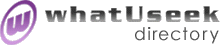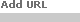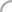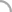SearchThe Directory The Webfor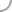SoftwareNew! Submit a site

Categories:
 Algebra *(43)Logic *(22)MATLAB (120) Calculators (41)Maple (20) Chaos and Fractals *(37)Mathcad (11) Combinatorics *(37)Mathematica (38)Number Theory *(59) Derive (9)Numerical Analysis *(31) Educational *(98)Potential Theory *(2)Simulators (2) Geometry *(20)Statistics *(88) Graphing (26)Typesetting (11)

whatUseek Collection Sites (submit a site ):

Give your site great placement in this category in as little as two business days!

whatUseek Directory Site Listings:

2D L-Systems - Two-dimensional L-systems

Adept Scientific - The Technical Computing People - Offers a broad range of personal computer products for mathematical, scientific and engineering applications.

Aepryus Software - Mathematics, Physics and Evolution Software.

Aptech Systems, Inc. - GAUSS - A data analysis environment based on the GAUSS Matrix Programming Language.

Atlantis Graphing/Math Software - Atlantis is powerful, user-friendly graphing/math software consisting of many features such as Graphing, Curve-Fitting, Statistics, Roots of Polynomials, Matrix Algebra, Integration, and much more.

Behavior Modeler - GrafiCalc allows users to simulate and solve a wide range of geometry based computational challenges.

CORE - Supports exact comparisons for expressions involving arithmetic and square roots, or if desired, faster inexact comparisons. Designed for exact geometric computation.

CPLEX - Mathematical programming software for resource optimization. Linear, mixed-integer and quadratic programming solvers.

Centre for Computing Information Systems and Mathematics at Athabasca University - Program information, online courses, examples of our students course projects, and research endeavors.

Chartwell-Yorke Mathematics Software and Books - Computer algebra mathematics software (DERIVE for Windows and Macsyma) PDEase numerical maths solver for partial differential equations, Cabri-Geometre II dynamic geometry software, TI-92 calculator, and books about their use in education and industry.

Clecom - Suppliers of symbolic, statistical and DSP software.

Curve Fit XYZ Computer Program - Curve fit program determines equation coefficients for functions y = f(x) and y = f(x,z). Polynomial, exponential and power form of the equation are included.

DataTrend Software - Grab It allows a scientist or engineer to digitize data without a graphic tablet. Loads any graph or chart and digitizes the data accurately and in real time.

Download VBMatrix mathematical software - VBMatrix is a scientific/mathematical software language using Visual Basic syntax, import ActiveX interpreter or compile to produce EXEs and DLLs for your application programs.

EasyPlot - Scientific Graphing and Data Analysis.

EclipseSoft - builders of Quadratica - Quadratica is a simple program for solving math problems using the quadratic equation, and systems of equations.

Euler - A numerical laboratory with a programming language, similar to Matlab.

Euromath Support Center - Distribution and support of Euromath software. Bratislava, Slovak Republic.

Flying Fox - Flying Fox is a "scientific data visualization" application.

[ 1 2 3 4 ]Help build the largest human-edited directory on the web.About   Help   Content Filter   Terms   Privacy Policy© 2018 whatUseek